# Question 1 (2 points) You have 10.0 g each of Na, C, Pb, Cu and Ne....Question 1 (2 points) You have 10.0 g each of Na, C, Pb, Cu and Ne. Which contains the largest number of moles? Question 1 options: Cu Pb с Na Ne Question 2 (2 points) Ionic bonds form because Question 2 options: Two atoms share their electrons. Two ions of the same charge are attracted to each other. Two sons of different charges are attracted to each other. Two atoms share their protons Question 3 (2 points) Determine the answer for the equation below with correct number of significant figures: 3.215 x 13.2 +0.218 = Question 3 options: 194.67 195 194.669 194.7 Question 4 (2 points) Oil floats on water. The most accurate reason for this is Question 4 options: Oil is less dense than water Oil is both less dense than water and immiscible (doesn't dissolve) in water Water is heavier than oil Oil is immiscible (does not dissolve) in water
A bag of flour contains 5.00 lbs of flour. You have 3.20 tons of flour. There are 2000lbs per ton How many bags of flour can you make? Question 5 options: 800 x 10 hp 800 x 10 hp 128x10 hp 1.3% 10 hops Question 6 (2 points) How many moles of lithium nitrate are theoretically produced if we start with 3.4 moles of Ca(NO3)2 and 24 moles of Li3PO4? Reaction: 3Ca(NO3)2 + 2Li3PO4 +6LINO3 + Ca3(PO4)2 Question 6 options: 23 Question 7 (2 points) Which of the following diagrams represents oxygen? 11111 10 Question 7 options: Question 8 (2 points) What do the alkali metals all have in common? Question 8 options: They all have similar physical properties They all from im. They all have the same number of Valence electrons
Question 9 (2 points) A precipitate is expected to be formed when an aqueous solution of sodium sulfate is added to an aqueous solution of Question 9 options: magnesium chloride potassium chloride ironi) chloride barium chloride Question 10 (2 points) I react Mg and HCl via the following reaction. Mga) + 2HCl(aq) +2H22) + MgCl2(aq) MgCl, is white as a solid and colorless if it dissolves in water. HCl is also colorless when it is dissolved in water If Mg is the limiting reagent, at the end of the reaction Question 10 options: hydrogen gas will continue to bubble through the solution. magnesium metal will be at the bottom of the flask. the solution will be completely transparent because the MgCl, is soluble and dissolved in the water the solution will be completely transparent but there will be solid MgCl, at the bottom of the flask. Adding more HCI will make the reaction start again and produce more product. Question 11 (2 points) Convert 0.666 moles of Be to grams Question 11 options: 13.5 g Be 0.063 g Be 6.00 g Be 4.5 x 10g Be 1.2 x 10g Be
What volume of gold (Au) contains a mass of 15.0 grams? (Density = 13.32 g/mL) Question 12 options: 00113 m. 14.9 m. 56.7 ml. 1.13ml. 888ml Question 13 (2 points) On the planet Glop there are several units to measure distance. 1.50 Snoofs - 8.00 Lulus, and 3.00 Lulus - 7.00 Homps. Convert 12.37 Snoofs to Homps. Question 13 options 154 28 5.4 Question 14 (2 points) Which one of the following has the most metallic character? Question 14 options: с H Mg Question 15 (2 points) How many grams of Sno, react with 12.00 grams of hydrogen? Use the equation below as part of your calculation SnO2 + 2H20 - 2H30 + Sno Molar masses: SnO,-150.71 g/mol H-2.02 g/mol H20-18.02 Sn-118.71 g/mol Question 15 options: 352 545 895.193 22380 1678 447655
Question 16 (2 points) What is the empirical formula of a compound this is comprised of 16.03 grams of sulfur (S) and 24.00 grams of oxygen? Question 16 options: SO SO SO SO, SO Question 17 (2 points) The diagrams below represent aqueous solutions of three acids (HX, HY, and HZ). Which acid is the weakest acid? Question 17 options: HX HY They are all the same strong Question 18 (2 points) Metals contract as they go from liquids to solids. Keeping in mind the definition of density, if a piece of solid metal is dropped in a vat of that same liquid metal what can you expect to happen before the metal melts. Question 18 options: It will sink Everything expands as it goes from liquid to solid. Evidence for this is that water put in a freezer will crack a tight container when it freezes It will have the same density as the liquid and be submerged but not on the bottom Metals can't melt and this is a trick question It will float
Question 19 (2 points) When the following equation is balanced, what is the coefficient for water if the coefficient for No, is one? N,H,+ NO.- HO+N, Question 19 options: One TWO Three Four Five Question 20 (2 points) What is the electron configuration for Ga? Question 20 options: 1:22:22p63.2353010424p1 1:22s22p63s23p64240104p! 1:22:22p6321106.04 1:22:22p63623646230104p1 Question 21 (2 points) An electron in a hydrogen atom absorbs aqua (green with a little blue) light in going from the n=2 to n=4 energy level. What is a possible color that could be absorbed if the electron goes from the n=2 to the n=5 energy level? Question 21 options: blue Infra-red orange Question 22 (2 points) Which of the following compounds is INSOLUBLE? Question 22 options: magnesium bromide lithium carbonate aluminum sulfide
Question 23 (2 points) How many neutrons are present in Ne-22? Question 23 options: 32 10 Question 24 (2 points) An atom is electrically neutral because Question 24 options: Neutrons balance the protons and cloctroms The numbers of protons and electrons are equal The numbers of protons and neutrons are equal. Nuclear forces stabilire the charges Question 25 (2 points) Which of the following is NOT an example of a physical property? Question 25 options: Water can free solid at 32 degrees Fahrenheit Water can form hydrogen and oxygen pas under diectrolysis conditions Liquid water can be om in het Solid ise can be very bile Question 26 (2 points) What charge is the element barium (Ba) most likely to form? Question 26 options: 2 . 1 Question 27 (2 points) What is the molecular formula of a compound with the empirical formula C,H, and a molar mass of 215.50 g/mol Question 27 options: CH CH CH. сна CH
Question 28 (2 points) Which one of the following atoms has the smallest radius? Question 28 options: Be с N Question 29 (2 points) Blue light travels faster in a vacuum than red light travels in a vacuum because blue light has more energy than red light. Question 29 options: False True Question 30 (2 points) You prepare a solution by adding a solid to water and shaking vigorously. After letting it settle, you observe solid at the bottom and a transparent solution above the solid. Which term properly describes the solutionabove the solid? Question 30 options: unsaturated insoluble saturated supersaturated Question 31 (2 points) You have an acid with a pH of 4.00. You have 2L of this acid. You add 3L more water to the solution. You would expect the pH of the new more dilute solution to Question 31 options: increase you can't tell without knowing whether the acid is weak ere strong stay the same decrease
Question 32 (2 points) Which two atoms represented by the symbols below are isotopes of oxygen? II. IV. X V x Question 32 options: IV & V III & V II & V I & III Question 33 (2 points) Which of the following items is a chemical property? Question 33 options: the odor of spearmint gun the cornered Corvete the melting and boiling point of water thetamishing of a copper statue Question 34 (2 points) A metal is in the shape of a box. The length of its sides are 3.0 yd, 2.0 yd, and 50 yd. What is its volume in ft. There are 3ft in a yard (yd) Question 34 options: 9.0 cubic feet 27 cubic feet 11 cubic feet 3.0 cubic feet 8lcubic feet Question 35 (2 points) How many moles of water are made from complete reaction of 2.2 moles of oxygen gas with excess hydrogen gas? Given the reaction: 2H2 +02-2H20 Question 35 options: 11 44 22
Question 36 (2 points) What is wrong with the reaction Zn2+ + Cu Cu + Zn Question 36 options: Zinc only forms + ions Mass is not conserved. Copper only forms + ions Charge is not conserved. Question 37 (2 points) Which energy sublevel is being filled by the elements Y through Cd? Question 37 options: Ss Sp Sf Sd Question 38 (2 points) Which of the following entities has the following electron configuration: 1s 2s22p Question 38 options: Ne 0 F N 0 Question 39 (2 points) What is the net ionic equation for the reaction of hydrochloric acid with potassium hydroxide if the molecular equation is HCI + KOH-HO, +KCI? Question 39 options: 2H2CH. +2+ + 2011.H20 K2 +2C1- H+CI-+k+ OH-H20K++CH- HCI KOH + H2OKCI H+ + OH-H20
Question 40 (2 points) What is the molar mass of Ca(NO3)2 ? Question 40 options: 32.07 g/mol 102.09 g/mol 68.94 g/mol 164,06 g/mol 45.95 g/mol Question 41 (2 points) 1. The shape shown below represents a(n) orbital: Question 41 options: 3 P Question 42 (2 points) The formula for aluminum oxide, a very stable ionic compound, is most likely to be Question 42 options: ALO, ΑΙΟ, ΑΙΟ AIO
Question 43 (2 points) What are the coefficients for the following reaction when it is properly balanced? _Na3PO4 + _Ba(NO3)2 → _NaNO3 + Ba3(PO4)2 Question 43 options: 2.1.1.3 6.1.3.2 2,3,6,1 Question 44 (2 points) Most of the mass of an atom is Question 44 options: In the electron cloud due to just the neutrons Evenly spread out In the nucleus Question 45 (2 points) A balanced chemical equation used to prepare ammonium carbonate, (NH4)2CO3, is: 2 NH3 + CO2 + H20 - (NH4)2CO3 Which choice of reactant quantities shown below would result in the greatest amount of ammonium carbonate being formed? Question 45 options: React 2 moles NH3, mole CO2, and I mole H20 React 2 moles NH3,8 moles CO2 and 8 moles H20 React 4 moles NH3. I mole CO2 and 2 moles H2O React 4 moles NH3,2 moles C02, and 2 moles H20 Question 46 (2 points) What is the molecular geometry if you have 3 single bonds and 1 lone pair around the central atom? Question 46 options: linear tetrahedral bent trigonal pyramidal
Question 47 (2 points) 1. What is the electronic geometry for ammonia, NH,? Question 47 options: bent/v-shaped linear trigonal planar trigonal pyramidal tetrahedral Question 48 (2 points) Which of the following is the correct Lewis structure for formaldehyde, CH,O? A. H-c=0-H :0 II B.H-C-H C.H-CEO-H :o: D. H-C-H Question 48 options: option A option B option option D
Question 49 (2 points) Which of the following is an observation as opposed to a hypothesis? Question 49 options: Light from the sun enters the earth's atmosphere less directly in winter than in spring making it colder. The earth is farther from the sun in the winter making it colder. The days are warmer in spring than winter on average. Persephone is in Hades during the winter and her mother mourns so it is colder than in the spring when she returns to earth and her mother rejoices. Question 50 (2 points) An isotope of an element forms an ion. The ion has a charge of -2 with a total of 10 electrons. The number of neutrons is 10. The ion is: Question 50 options: 22Na2 B-2 20Ne? 180-2 1002

This Homework Help Question: "Question 1 (2 points) You have 10.0 g each of Na, C, Pb, Cu and Ne...." No answers yet.

We need 10 more requests to produce the answer to this homework help question. Share with your friends to get the answer faster!

0 /10 have requested the answer to this homework help question.

Once 10 people have made a request, the answer to this question will be available in 1-2 days.
All students who have requested the answer will be notified once they are available.

#### Earn Coin

Coins can be redeemed for fabulous gifts.

Similar Homework Help Questions
• ### DATE 1. How many moles of Na are there in 47.3 g of Na? 2. Calculate...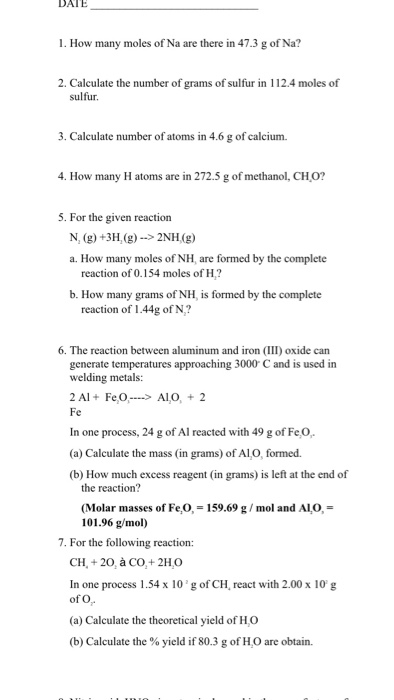DATE 1. How many moles of Na are there in 47.3 g of Na? 2. Calculate the number of grams of sulfur in 112.4 moles of sulfur. 3. Calculate number of atoms in 4.6 g of calcium. 4. How many H atoms are in 272.5 g of methanol, CH0? 5. For the given reaction N, (g) +3H,(g)->2NH(g) a. How many moles of NH, are formed by the complete reaction of 0.154 moles of H? b. How many grams of NH,...

• ### Question 4 1 pts Which of the following mole ratios CANNOT be derived from the reaction 2 502 (g) + O2 (g) + 2 H2...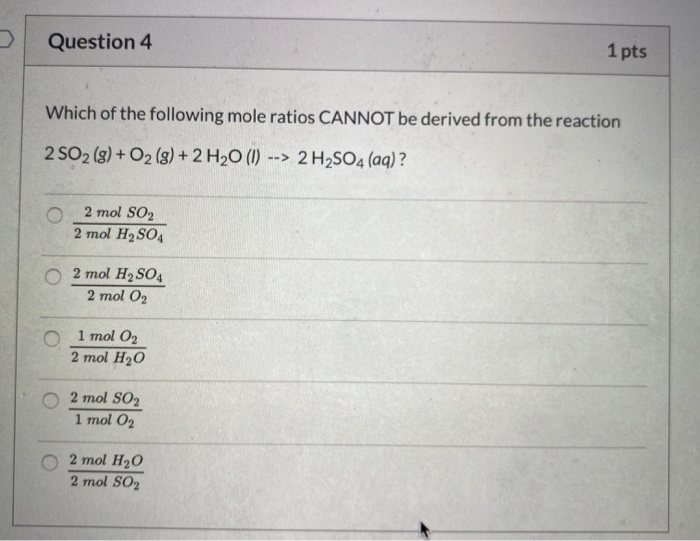Question 4 1 pts Which of the following mole ratios CANNOT be derived from the reaction 2 502 (g) + O2 (g) + 2 H20 (1) --> 2 H2SO4 (aq) ? 02 mol SO2 2 mol H2SO4 2 mol H2SO4 2 mol O2 0 1 mol O2 2 mol H20 O 2 mol O2 1 mol O2 2 mol H20 2 mol SO2 Question 1 1 pts What do the coefficients in a balanced equation tell us? Mark ALL that...

• ### H2SO4 Heat нас 2 CH-OH H₂ CH-0 2 H3C-OH + H2C CR A C | CH3...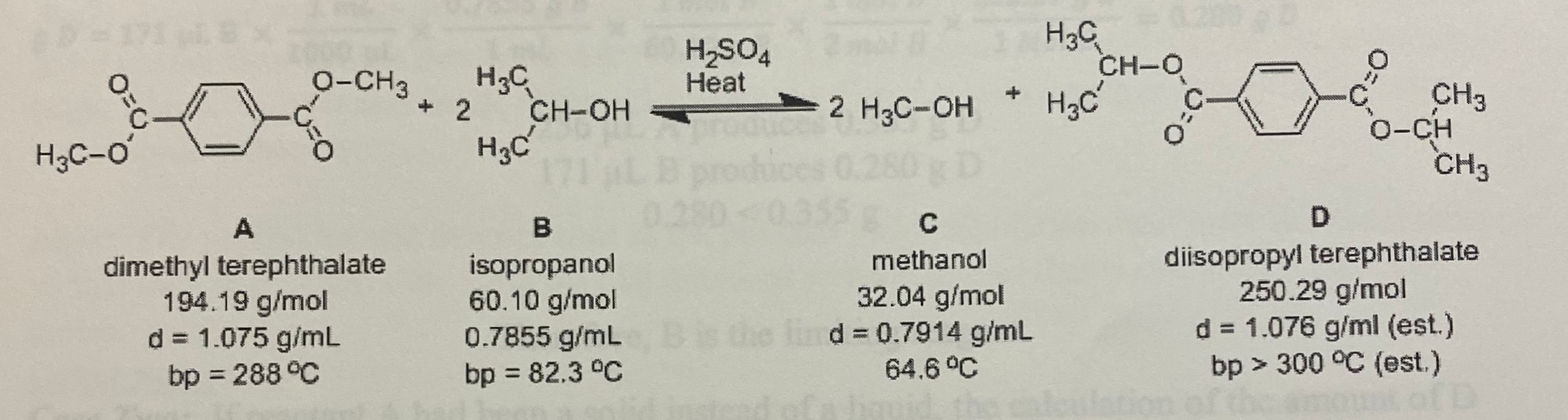H2SO4 Heat нас 2 CH-OH H₂ CH-0 2 H3C-OH + H2C CR A C | CH3 0-CH. нас CH3 dimethyl terephthalate 194.19 g/mol d = 1.075 g/mL bp = 288°C isopropanol 60.10 g/mol 0.7855 g/mL bp = 82.3 °C methanol 32.04 g/mol d = 0.7914 g/mL 64.6 °C diisopropyl terephthalate 250.29 g/mol d = 1.076 g/ml (est.) bp > 300 °C (est.) Problem Two. Start over. This time assume A is a solid and 423.6 mg of A was measured...

• ### From the following balanced equation, 4 NH3(g) + 50,(9) — 4 NO(g) + 6H20(1) how many...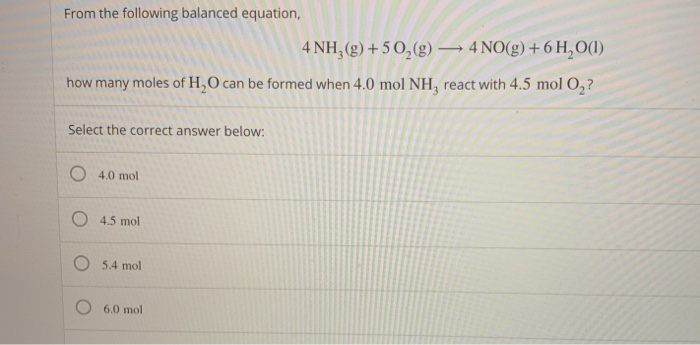From the following balanced equation, 4 NH3(g) + 50,(9) — 4 NO(g) + 6H20(1) how many moles of H,O can be formed when 4.0 mol NH, react with 4.5 mol 0,? Select the correct answer below: O 4.0 mol O 4.5 mol O 5.4 mol O 6.0 mol What is the mass, in grams, of 3.80 moles C2H6? Provide your answer below: When 5.58 g 0, react by the following balanced equation, 3.28 g H,O are formed. What is the...

• ### Reaction 1: Use in question 3 Pb(NO3)2 (aq) + Kl (aq) → KNO, (aq) + Pblz...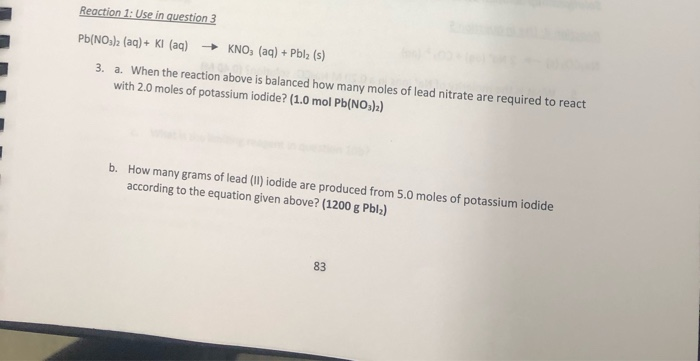Reaction 1: Use in question 3 Pb(NO3)2 (aq) + Kl (aq) → KNO, (aq) + Pblz (s) 3. a. When the reaction above is balanced how many moles of lead nitrate are required to react with 2.0 moles of potassium iodide? (1.0 mol Pb(NO3)2) b. How many grams of lead (II) iodide are produced from 5.0 moles of potassium iodide according to the equation given above? (1200 g Pblz)

• ### 1. (9 points) Balance the following reactions: H3PO4(1) + HCIE) C4H3 + O2 CO2 + H2O...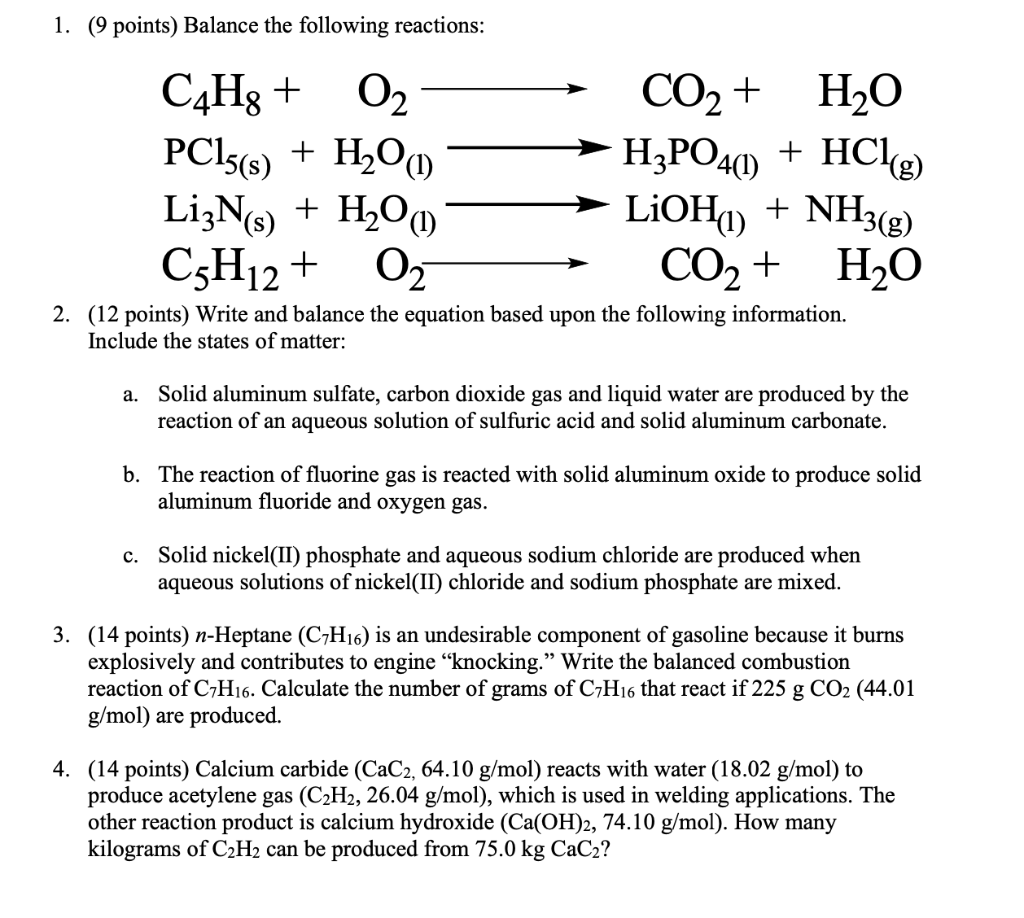1. (9 points) Balance the following reactions: H3PO4(1) + HCIE) C4H3 + O2 CO2 + H2O PC15(s) + H2O LizNs) LiOH) + NH3(g) C3H12 + O2 CO2 + H2O 2. (12 points) Write and balance the equation based upon the following information. + H2O(1) Include the states of matter: a. Solid aluminum sulfate, carbon dioxide gas and liquid water are produced by the reaction of an aqueous solution of sulfuric acid and solid aluminum carbonate. b. The reaction of fluorine...

• ### are these right? im having a hard time on number 2 a-c Experiment 9-Post-lab Questions 1....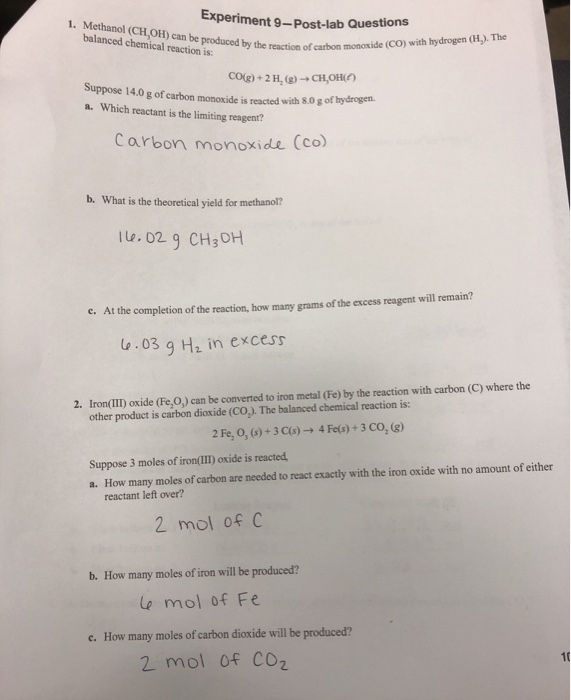are these right? im having a hard time on number 2 a-c Experiment 9-Post-lab Questions 1. Methanol (CH,OH) can be produced by the reaction o balanced chemical reaction is by the reaction of carbon monoxide (CO) with hydrogen (H). The CO(g) + 2 H (g)-CH2OH) Suppose 14.0 g of carbon monoxide is reacted with 8.0 g of hydrogen 3. Which reactant is the limiting reagent? Carbon monoxide (co) b. What is the theoretical yield for methanol? 16.02 g CH₂OH c....

• ### 9 b) the reaction between aqueous solutions of cobalt(III) nitrate and sodium hydroxide to form aqueous sodium nitra...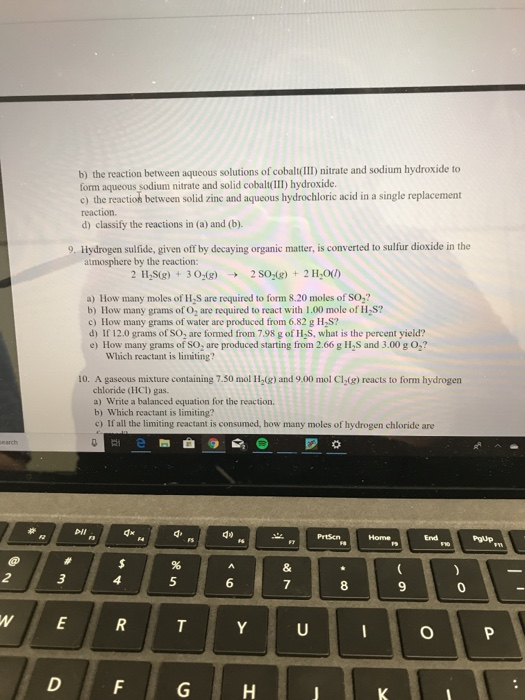9 b) the reaction between aqueous solutions of cobalt(III) nitrate and sodium hydroxide to form aqueous sodium nitrate and solid cobalt(III) hydroxide. c) the reaction between solid zinc and aqueous hydrochloric acid in a single replacement reaction. d) classify the reactions in (a) and (b). 9. Hydrogen sulfide, given off by decaying organic matter, is converted to sulfur dioxide in the atmosphere by the reaction: 2 H S(g) + 30 (g) 2 SO (g) + 2 H2O() a) How many...

• ### *please solve using dimensional analysis, thank you* 4. When liquid 2-chloro-2-methylpropane, CaHsCl, is added to ethanol...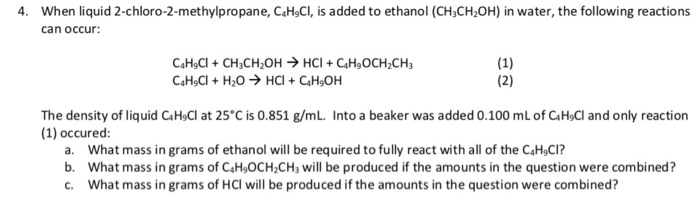*please solve using dimensional analysis, thank you* 4. When liquid 2-chloro-2-methylpropane, CaHsCl, is added to ethanol (CH3CH2OH) in water, the following reactions can occur: САн,Сі + CH,сH,0н нсі + С.Н,осн,CH, C&HCI + H20 HCI CH,OH (1) (2) The density of liquid CaHsCl at 25'C is 0.851 g/mL. Into a beaker was added 0.100 mL of CaHsCl and only reaction (1) occured: a. What mass in grams of ethanol will be required to fully react with all of the C4HCI? b....

• ### is the pH and pOH of the acid solution with [H'] = 0.38 M? What is...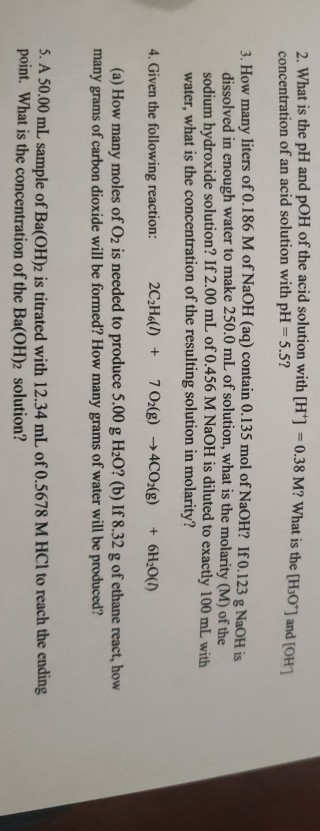is the pH and pOH of the acid solution with [H'] = 0.38 M? What is the [H30") and TOH concentration of an acid solution with pH = 5.5? 3. How many liters of 0.186 M of NaOH(aq) contain 0.135 mol of NaOH? If 0.123 g NaOH is dissolved in enough water to make 250.0 mL of solution, what is the molarity (M) of the sodium hydroxide solution? If 2.00 mL of 0.456 M NaOH is diluted to exactly 100...